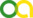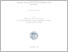Christou, Christos (2019). Non-Equilibrium Stochastic Models: Random Average Process and Diffusion with Resetting. PhD thesis, Universität zu Köln.Preview
PDF
thesis4.pdf

## Abstract

In this work we consider two non-equilibrium models, the random average process and the diffusion with resetting. Both of these models have very interesting features and are relevant for a variety of fields. The random average process is defined on one-dimensional periodic lattice and equipped with nearest neighbor interaction. The state of the system is described by continuous variables that are called masses. We focus here on totally asymmetric and discrete time dynamics. This means that at each time-step an asymmetric mass exchange between neighboring sites takes place. The fraction of the transported masses is defined by the probability density function $\phi$ which we call fraction density. We start by introducing a special case of the random average process with state-dependent fraction density, the truncated random average process. We will give here a short overview over the different properties of three distinct truncated models with finite lattice size. A new kind of random average process is introduced in this work that is characterized by the fact that only sites, who have a mass that is above a certain cutoff, can give a fraction of this mass to their neighboring sites. A special property of this model is the existence of a steady state that is an absorbing state. We determine analytically the exact form of this absorbing state in the thermodynamic limit by a canonical ensemble approach. This exact form also holds for systems that are partial asymmetric. Furthermore the provided single site distribution function is a good approximation for finite size systems. The second class of models studied in this work are diffusive search processes with resets. The fact that random resetting may reduce the mean duration of a diffusive search process is rather intriguing. Here we re-evaluate this positive effect of resetting for the special case of stochastic processes that take place in bounded domains. It will be shown here that for a diffusion process taking place in one-dimensional domain with reflecting boundaries an optimal resetting rate can be found. The exact value of this optimal value is provided by the solution to the Laplace Transform of the Fokker-Planck equation. Additionally we are in the position to calculate the non-equilibrium steady state for this process. Finally we consider a diffusion search process inside a two-dimensional circular domain that switches between two phase of motion while also having the property of resetting to the initial position. We calculate the mean time to absorption for several variations of this procedure by determining the corresponding survival probability. From the derived results we can define for which parameter values resetting is still beneficial for this kind of diffusive search.

Item Type: Thesis (PhD thesis)
Creators:
CreatorsEmailORCIDORCID Put Code
Christou, Christoschristoschristou@ymail.comUNSPECIFIEDUNSPECIFIED
URN: urn:nbn:de:hbz:38-95255
Date: 16 April 2019
Language: English
Faculty: Faculty of Mathematics and Natural Sciences
Divisions: Faculty of Mathematics and Natural Sciences > Department of Physics > Institute for Theoretical Physics
Subjects: Physics
Uncontrolled Keywords:
KeywordsLanguage
Non-Equilibrium PhysicsEnglish
DiffusionEnglish
Large Deviation TheoryEnglish
Date of oral exam: 4 September 2018
Referee:View Item